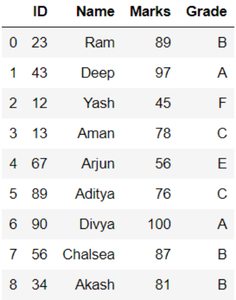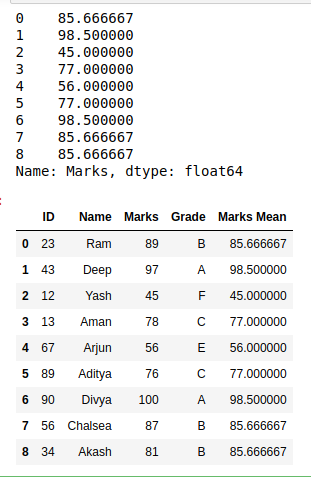GeeksforGeeks App
Open AppBrowser
Continue

# How to Add Group-Level Summary Statistic as a New Column in Pandas?

In this article, we will learn how to Add Group-Level Summary Statistic as a New Column in DataFrame Pandas. This can be done by using the concept of Statistic mean, mode, etc. This requires the following steps :

1. Select a dataframe
2. Form a statistical data from a column or a group of columns
3. Store data as a series
4. Add the series in dataframe as a column.

Here, we take a dataframe, The Dataframe consists of student id, name, marks and grades. Let’s create the dataframe

## Python3

 `# importing packages``import` `pandas as pd`` ` `# dictionary of data``dct ``=` `{``'ID'``: {``0``: ``23``, ``1``: ``43``, ``2``: ``12``,``              ``3``: ``13``, ``4``: ``67``, ``5``: ``89``,``              ``6``: ``90``, ``7``: ``56``, ``8``: ``34``},``        ` `       ``'Name'``: {``0``: ``'Ram'``, ``1``: ``'Deep'``,``                ``2``: ``'Yash'``, ``3``: ``'Aman'``,``                ``4``: ``'Arjun'``, ``5``: ``'Aditya'``,``                ``6``: ``'Divya'``, ``7``: ``'Chalsea'``,``                ``8``: ``'Akash'``},``        ` `       ``'Marks'``: {``0``: ``89``, ``1``: ``97``, ``2``: ``45``, ``3``: ``78``,``                 ``4``: ``56``, ``5``: ``76``, ``6``: ``100``, ``7``: ``87``,``                 ``8``: ``81``},``        ` `       ``'Grade'``: {``0``: ``'B'``, ``1``: ``'A'``, ``2``: ``'F'``, ``3``: ``'C'``,``                 ``4``: ``'E'``, ``5``: ``'C'``, ``6``: ``'A'``, ``7``: ``'B'``,``                 ``8``: ``'B'``}``       ``}`` ` `# create dataframe``df ``=` `pd.DataFrame(dct)`` ` `# view dataframe``df`

Output:Now, we will find the group level statistics summary using the above approach.

## Python3

 `# make a series``new_column ``=` `df.groupby(``'Grade'``).Marks.transform(``'mean'``)`` ` `# view new series``print``(new_column)`` ` `# add column in dataframe``df[``"Marks Mean"``] ``=` `df.groupby(``'Grade'``).Marks.transform(``'mean'``)`` ` `# view modified dataframe``print``(df)`

Output:My Personal Notes arrow_drop_up